## research papersBIOLOGICALCRYSTALLOGRAPHY
ISSN: 1399-0047
Volume 62| Part 1| January 2006| Pages 96-101

## Some aspects of qu­antitative analysis and correction of radiation damage

aUniversität Konstanz, Fachbereich Biologie, M647, D-78457 Konstanz, Germany
*Correspondence e-mail: kay.diederichs@uni-konstanz.de

(Received 13 April 2005; accepted 3 October 2005)

Radiation damage is the major source of systematic error in macromolecular data collected at third-generation synchrotron beamlines. In this paper, a simple way of analysing data for radiation damage is proposed and shown to give results that are easy to interpret. Results of correction of radiation damage obtained with an exponential decay function as implemented in XSCALE (from the XDS package) are shown, and aspects of the mathematical treatment of radiation damage, as well as experimental requirements for the correction and utilization of radiation damage are discussed. Furthermore, a method for quantifying the coverage and evenness of sampling of rotation range is proposed.

### 1. Introduction

In recent years it has become clear that radiation damage of macromolecular X-ray data collected at synchrotrons is the major systematic source of error for most of the data sets measured. Ionization events in the crystal can conceptually be divided into two components: primary radiation damage directly and unspecifically affects all atoms in the crystal, but has its greatest effect on the occupancy (and sometimes position) of heavy atoms (sulfur and metals) because of their comparatively large absorption cross section (reviewed in Garman, 2003). Secondary radiation damage arises from highly reactive free radicals and photoelectrons produced by the incident radiation. Even at cryotemperature (near 100 K) the photoelectrons diffuse through the crystal, although at much reduced rates, and target susceptible groups in macromolecules.

Taken together, these events produce both unspecific, random damage and specific, localized changes of the macromolecular structure. Random damage of atoms leads to disorder in the crystal and ultimately to deterioration of the diffracting power of the crystal. Henderson (1990) estimated that the maximum dose tolerated by a crystal amounts to about 2 × 107 Gy, a dose reached at third-generation undulator beamlines within minutes of irradiation with an unattenuated beam.

The specific changes caused by radiation damage have important consequences for phasing, refinement and interpretation of macromolecular structures. The loss of high resolution brought about by unspecific radiation damage reduces the amount of information in refined structures, and structural changes owing to irradiation might lead to the wrong conclusions concerning functional aspects of protein structure. Experimental phasing of macromolecules depends on the presence of naturally available or experimentally introduced heavy atoms bound at specific sites of the structure. Radiation damage reduces the occupancy and sometimes location of exactly those atoms which are used for phasing, and, in principle, results in a breakdown of the theory used to derive phases from the small differences of structure factors in single/multiple anomalous dispersion (SAD and MAD) or single/multiple isomorphous replacement (SIR and MIR) experiments, because theory assumes that the contribution of the heavy-atom substructure on the structure factors can be calculated from its time-independent model, and does not depend on the time (or rather, dose) when a reflection was recorded during the experiment.

Experimentally, secondary radiation damage is greatly reduced by cryocooling. Additionally, radical scavengers like ascorbate have been shown to further reduce secondary radiation damage (O'Neill et al., 2002; Garman, 2003). Strong attenuation and short exposure times are commonly used to find a compromise between data quality and radiation damage. At the data reduction stage, an artificial temperature factor is employed to correct the average radiation damage, both as a function of dose and of resolution.

Quantitative ways of assessing radiation damage have been sought, but neither the changes in cell parameters, nor those in mosaicity or a number of other parameters investigated have been found to be reproducible among crystals even of the same species (Murray & Garman, 2002; Ravelli et al., 2002). Correction of specific radiation damage has been shown to be effective at the level of the raw, unmerged intensity data, thus exploiting the redundancy (multiplicity) of observations of the unique reflections during data reduction and scaling (Diederichs et al., 2003). Alternatively, correction was achieved by refinement of heavy-atom parameters as a function of dose against multiple observations during phasing (Schiltz et al., 2004).

Here, aspects of the former correction are discussed, and a way of analysing data with respect to radiation damage is suggested and explored. Furthermore, indicators for the characterization of coverage and sampling of a data set's rotation range, and therefore also of its radiation damage, are proposed, which may be used to optimize data-collection strategy with respect to radiation damage and its correction.

### 2. Methods

The method suggested by Diederichs et al. (2003) requires and exploits redundancy (multiplicity of observations belonging to each unique reflection) to partially correct radiation damage. The basic idea is that the dose-dependent changes in electron density result in non-random, dose-dependent values of the structure factors, which can be approximated by a low-order function fitted to all observations of each unique reflection. The underlying physical model is the decomposition of the electron density into two parts, one that is constant, and one that depends on dose and can be used to model the (specific and unspecific) radiation damage.

It is worth noting that a zero-order model is fitted to all observations of a unique reflection in the traditional scaling model which does not explicitly take radiation damage on individual reflections into account, thereby neglecting the information contained in the dose-dependency of the intensities.

#### 2.1. Models for radiation damage at the level of unique reflections

Although radiation damage is a local phenomenon, its manifestation in the measured intensities involves the average over a large number of unit cells in the crystal. These intensities can therefore be considered slowly varying functions of the dose, at least over the range of doses commonly employed for synchrotron measurements of protein crystals. Low-order functions can therefore be used to model the radiation-induced change of intensities.

As particularly simple functions, a quadratic, a linear and an exponential model are defined and characterized below.

The constant, radiation-insensitive part of the dose dependent electron density ρ(x, y, z; d) can be denoted as ρc(x, y, z), and the radiation-sensitive part, which depends on the dose d, is denoted as ρv(x, y, z; d), thus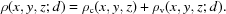If we assume that ρv(x, y, z; d) is linear in the dose, we can write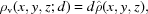where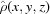is the change in electron density of the radiation-susceptible part of the structure upon irradiation with a unit dose. In reciprocal space, omitting the indices hkl of the structure factors for brevity, the structure factor corresponding to the constant ρc(x, y, z) is the dose-independent F, and the structure factor corresponding to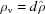is dG. Then, the total structure factor h of a reflection as a function of dose is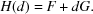The total intensity yquad(d) = |H(d)|2 is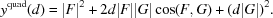yquad(d) therefore depends on two parameters, |G| and the cosine of the angle between F and G, which need to be determined by least-squares fitting to the observed intensities, in addition to the zero-dose intensity |F|2. In this way, provided that the assumption of linearity in dose holds, the observations of a dose-dependent intensity may be fully described, except for noise. However, the least-squares determination of the two parameters from the observations is not straightforward because of the restrictions on the possible values of the cosine.

In the presence of unit-cell changes induced by radiation damage, the sampling of the fourier transform of the macromolecule differs between the observations of a unique reflection. In this case, the quadratic model is not justified, and the coefficients of the quadratic model do not provide a valid mathematical interpretation of the changes in the electron density.

##### 2.1.2. Linear model

Although the quadratic model approach shows the mathematical background and underlying assumptions, a simplified approach appears to be adequate in practice. The simplest approach is a linear function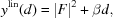where β is an empirical decay factor' (or damage factor') without direct physical significance, which can be determined from a linear fit to the observations of each unique reflection. This was implemented by the author in the program 0-dose (Diederichs et al., 2003) for the general case, where β is determined from observations belonging to several data sets. A shortcoming of this model is that the correction may result in negative extrapolated intensities.

##### 2.1.3. Exponential model, and implementation in XSCALE

A better approximation can be expected from an exponential function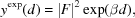where β is again a decay factor' which is determined from a nonlinear fit to the observations of each unique reflection. This model has the advantage that the theoretical values and their second derivative are positive, as in the case of the quadratic model.

The algorithm was implemented in the program XSCALE (Kabsch, 2004), again for the general case where β is determined from observations belonging to several data sets. Furthermore, XSCALE determines by least-squares fitting two parameters per data set, both of which can also be input manually. The first of these (STARTING_DOSE') takes into account the dose that the crystal has absorbed before the start of the data set, and the second (DOSE_RATE') is a conversion factor from frame number to dose, which could be used in the case of data sets collected at different wavelengths or with different exposure times. In the case of only one data set, this parameter is set to one.

XSCALE extrapolates to zero dose, and STARTING_DOSE is by default zero for the first data set. If the user wants to extrapolate or interpolate intensities to any other value of the absorbed dose, a different STARTING_DOSE can be input. A negative value for STARTING_DOSE leads to interpolation within the dose range of the data set as long as the absolute value is less than the highest frame number difference within the data set.

#### 2.2. Analysis of decay

Radiation damage leads to a dose-dependent deviation of intensities from the (theoretical) intensity at zero dose. On average, the absolute difference becomes larger with larger difference in dose (see e.g. Table 2 in Ravelli & McSweeney, 2000, Table 1 in Banumathi et al., 2004and Table 5 in Weiss et al., 2005). A simple way to analyse radiation damage is a plot of fractional differences, which are calculated in analogy to the usual R factor, as a function of the separation of the contributing observations in framenumber (dose) space. We define a decay R factor'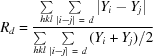such that the differences of the observed intensities Y of the unique reflection hkl with centroids on frames i and j contribute towards Rd at d = |ij|. As all pairs of observations of each unique reflection hkl contribute to this R factor as a function of framenumber difference', a relatively smooth function is obtained which gives a quantitative way of assessing radiation damage based on the measured data, without reference to any theoretical model of radiation damage.

Contrary to Rsym (Diederichs & Karplus, 1999), Rd does not depend on the multiplicity, because only pairwise comparisons are performed.

Plots of Rd (Fig. 1) by resolution shell can be routinely used to check data sets for radiation damage. A positive slope indicates significant radiation damage (Fig. 1a). After radiation-damage correction, the slope should be around zero (Fig. 1b).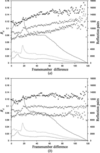Figure 1 R factor as a function of frame number difference (Rd), shown for a test data set. The crystal (Köhler et al., 2004) has space group P6522; each frame covers one degree of rotation. (a) No radiation damage correction performed in XSCALE. (b) same data, but after radiation damage correction in XSCALE. +', all data; ×', data in 10–5 Å resolution range; *', data in 3.5–3 Å resolution range. The lines show the number of reflection pairs contributing to each data point for all data (solid), data in 10–5 Å resolution range (long dashes); data in 3.5–3 Å resolution range (short dashes).

The difference (without radiation-damage correction) between R factors at low (Rd,low) and high (Rd,high) frame number differences can be used to quantify the damage. To enable the comparison of radiation damage between different proteins, or different crystal forms or growth conditions of the same protein, we may in the simplest case calculate a resolution-dependent quantity srd' considered to be a susceptibility for radiation damage' as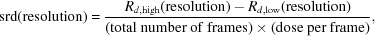in units of R factor increase per dose.

#### 2.3. Sampling of rotation range and radiation damage

For a given crystal orientation and geometry of the diffraction experiment, software is usually available to plan data collection, in order to obtain sufficient completeness of data and multiplicity of observations. Completeness is important to collect all available information about a crystal structure, and multiplicity is required to allow scaling and outlier rejection in the traditional scaling model. When radiation damage correction should be performed, multiplicity is also required to determine the decay factor(s) of each unique reflection.

If the dose per image is constant (or slowly varying), the radiation damage of a crystal is proportional to the amount of its exposure to the beam, and therefore to the fraction of its rotation in the data set's rotation range. In order to allow accurate interpolation of intensities to dose values within the data set's rotation range and possibly slightly beyond it, it is important to both cover as much as possible of the whole rotation range of the crystal with the observations, and to collect the observations in equal intervals, rather than to collect them (for example) in pairs because of the alignment of a symmetry axis with the axis of rotation. If either of these requirements is not met, the multiplicity cannot be expected to be a good indicator for the possibility of interpolation and extrapolation.

According to the author's knowledge, definitions of parameters quantifying these effects have not been given in the literature. Possible definitions are given below.

##### 2.3.1. Coverage of rotation range

A straightforward way to define the coverage C of rotation space for a single unique reflection is to calculate the fraction of total rotation range of the data set (φmaxφmin) covered by its n observations. If the crystal is rotated around the φ axis, the observations of a reflection are measured at φ1φn, where the index 1 refers to the first observation, and n to the last observation. We define for each reflection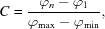which is a number between zero, if only one observation is made, and one, if first and last observation are measured at the start and end of data set. Intermediate values indicate an incomplete coverage of total rotation space in an obvious way. The average value of C over all unique reflections in a data set could be a parameter that should be useful to optimize, together with the resulting completeness, as a function of the starting φmin value of a data set.

##### 2.3.2. Evenness of sampling of rotation range

A possible way of calculating the evenness of sampling of rotation range', and therefore of the evenness of sampling of radiation damage, for individual unique reflections is the following. Again, we assume that n observations of a given unique reflection cover a rotation range φnφ1. We can then calculate the rotation intervals Δi = φiφi−1 for i > 1 and define the sampling entropy H as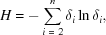with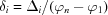, and the evenness E of sampling as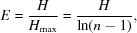with E defined as 0 if n ≤ 2.

This usage of the entropy H parallels that in other natural sciences, and results in an evenness E of sampling with a value of zero in the case of less than two intervals, and with a value of one when all intervals (n > 1) are of the same size. Intermediate values result if the intervals are of unequal size.

Again, the average value of E over all unique reflections in a data set could be a parameter that should be useful to optimize, together with the resulting completeness and coverage, as a function of the starting value φmin of a data set. As it is likely that not all three parameters are optimal for the same φmin value, a compromise should be sought depending on the purpose of data collection.

### 3. Results and discussion

In §§3.1 to 3.4, aspects of quantification and correction of radiation damage at the level of individual reflections by different models are shown and discussed. The corrections are assumed to be done in addition to the usual artificial temperature factor correction of the zero-order model. In §§3.5 and 3.6, further aspects are discussed which relate to various data collection parameters.

#### 3.1. Analysis of radiation damage by the Rd plot

The Rd plot has been used to detect radiation damage in a number of projects. Fig. 1shows Rd plots of data measured at the SLS (Swiss Light Source, Villigen, Switzerland) without and with radiation-damage correction (exponential model). Without radiation-damage correction of individual reflections, the slope of Rd is found to be higher for high-resolution shells than for low-resolution reflections. After correction, the slope is close to zero in all resolution ranges.

Within the limited experience obtained so far, the features seen in Fig. 1are common to the projects where these calculations were made. As the slopes of Rd appear to be reproducible across data sets from crystals of the same drop, and qualitatively similar for high- and low-resolution shells, it can be expected that Rd gives a more robust quantitative estimation of radiation damage than changes in e.g. cell volume or mosaicity, and is also applicable for low-resolution data.

#### 3.2. Modelling of radiation damage at the level of unique reflections

Obviously, if the constant part of the electron density as well as the changes in substructure upon irradiation were known, the radiation-induced changes in the intensities of the reflections could be directly calculated. This might be useful for high-resolution refinement of macromolecules, if a dose-dependent protein structure is to be modelled. In the case of heavy-atom substructure refinement this has been explicitly (Schiltz et al., 2004) or implicitly (Weiss et al., 2004) carried out to obtain better experimental phases. Likewise, if the constant part is known, the changes in the radiation-susceptible parts can be analysed by interpolation (with any of the low-order models) to a number of snapshots along the kinetic coordinate of radiation damage (Diederichs et al., 2003; Wang & Ealick, 2004).

#### 3.3. Choice of model

The quadratic model, if applicable, gives insight into the mathematical side of the problem. If the radiation-sensitive substructure (which might often coincide with those atoms that produce the dispersive/isomorphous or anomalous signal) is known and the observations of each unique reflection can be measured with high accuracy, then the absolute value of the phase difference between the structure-factor contribution arising from the protein and that from the substructure could be directly determined (Banumathi et al., 2004).

However, both assumptions underlying the quadratic model can often not be considered to be fulfilled in practice, as the kinetics are unlikely to follow a linear dose-damage relationship. Furthermore, the determination of two additional parameters instead of one (in the linear and the exponential model) increases the risk of overfitting of the experimental information. On the other hand, the one-parameter models lack the physical interpretation.

#### 3.4. Robust estimation at 1/4-dose and 3/4-dose

In this situation, it is useful to discuss a feature common to all least-squares fits with low-order functions. If we assume that the true' dose-dependency is itself an unknown low-order function resulting in the observed values Y(d), then the task of each of the possible models y(d) is to approximate the unknown function as well as possible in a least-squares sense. This will result in a small number of continuous ranges (1 or 2) where the function values y(d) are bigger than those of Y(d). Likewise, in a small number of continuous ranges (2 or 1) the function values y(d) will be smaller than those of Y(d). The error of the approximation is biggest at the ends of the dose interval, and is smallest where Y(d) and y(d) intersect. For all the low-order models, the points of intersection are close to 1/4 and 3/4, irrespective of the model used (Fig. 2).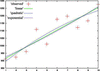Figure 2 Example of noisy data points (marked by `+') fitted by a linear (green), a quadratic (blue) and an exponential function (purple). Near 1/4 and near 3/4 of the range, the values of the functions used for fitting coincide.

We can therefore conclude that the error made by the approximation of the unknown dose-dependency is greatest when an extrapolation is performed to zero or maximum dose, and is smallest when interpolating to 1/4 or 3/4 of the maximum dose. Near the latter points, choice of a different low-order model does not result in a substantially changed interpolated value for the intensity. Therefore, the choice of the model does not matter much, if the interpolation is performed at these points.

#### 3.5. Effect of irradiated volume

The applicability of all mathematical models, and therefore of the computational correction of radiation damage, depends on whether the crystal is uniformly irradiated during the experiment. If the size of the crystal perpendicular to the spindle axis is bigger than the beam, then fresh parts of the crystal enter the beampath during rotation of the spindle; only the center of the crystal obtains the full dose (Fig. 3). The resulting radiation damage can in principle be described as a superposition of radiation effects at different doses. Clearly, no simple low-order model can account for this situation.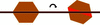Figure 3 Schematic diagram showing the trace of the beam through the crystal at the start of data collection (left), and after two exposures (right). Those parts of the crystal damaged by the beam are indicated in orange. The view is along the spindle axis.

For a data set measured like this, the changes in intensities owing to radiation damage are biggest at the beginning of the data set, when the center of the crystal still contributes to the observed structure factors. Later in the data set, the center of the crystal is amorphous and a dynamic equilibrium situation may be reached which is not accessible to radiation-damage correction, or does not even require any. Finally, after 180° rotation, no unirradiated crystalline material is available to enter the beampath, and another sudden change of diffraction properties happens – the resulting intensities represent diffraction arising solely from radiation-damaged protein, and a different dynamic equilibrium results. This repeats itself with a period of 180°, which might have implications for RIP (Ravelli et al., 2003) experiments. Clearly, no matter whether radiation-damage correction is performed or not, this also means that data sets should be scaled in batches of 180°.

Experiments handling this effect of the irradiated volume properly fall into two classes: either the beam is much smaller than the crystal, or the beam is about as big as the crystal. In the first case, the dynamic equilibrium is reached soon (provided that the flux is high enough), the first few frames may be discarded, and from then on mostly fresh protein contributes to the measured intensities. This might be a desirable situation at a high-flux undulator beamline, when a big crystal is available; intensites which are little affected by radiation damage can then be collected. Computationally, the situation may be characterized with an Rd plot; in the dynamic equilibrium case the slope of Rd should be smaller for ranges of data up to 180° than for a small data range near the beginning of data collection.

If a big crystal is not available or a very fine beam cannot be produced, an attempt should be made to match the size of the beam to that of the crystal (in the direction perpendicular to the spindle) by adjustment of optical elements (e.g. focus, slits). In this case, the measured intensities may be extrapolated or interpolated with any of the low-order functions discussed above.

#### 3.6. Coverage and sampling of rotation and dose space

At the current state of knowledge, it is still unknown which multiplicity of reflections is required to allow a reliable correction of radiation damage at the level of individual reflections. One important aspect is that the dose space should be sampled evenly by the observations, in order to accurately determine the parameter(s) of the decay model, and to allow inter- and extrapolation. For example, if all observations of a unique reflection are measured closely together, and therefore correspond to a similar dose, the sampling is poor, whereas an even sampling would result if the observations were spread out in the rotation (or rather, dose) range covered by the experiment.

The formalism given in §2.3.1and §2.3.2can be used to assess the coverage and evenness of sampling of rotation range, and therefore of dose space, for each unique reflection. Both coverage C and evenness E are numbers between 0 and 1. An evenness of 1 corresponds to the most even sampling, with equal rotation angle (dose) intervals for each of the observations. If all reflections occur in pairs (e.g. because of a certain alignment of symmetry elements with the rotation axis) and these pairs are evenly spaced, the evenness drops from one to ln(n/2 − 1)/ln(n − 1). The case of E = 0 corresponds to the situation of poor sampling (zero or one interval).

The average coverage and evenness of all reflections in a data set are therefore useful indicators which could be optimized or at least monitored by software which is designed to plan the data collection based on a given geometry of the diffraction experiment.

At the level of individual reflections, complete cross-validation (Diederichs et al., 2003) or a different statistical significance test based on the actual data could be performed to assess whether inter- and extrapolation of intensity is going to be reliable or not. An implementation of a statistical significance test is available in XSCALE (keyword 0-DOSE_SIGNIFICANCE_LEVEL).

#### 3.7. Concluding remarks

Radiation damage analysis and correction is still a new field of research. The aspects and indicators discussed here are hoped to advance the understanding of some important parameters in a diffraction experiment, to foster software improvements and ultimately to result in better data and new ways to obtain insight into physical, chemical and biological phenomena.

### Acknowledgements

The author would like to thank Drs R. Ravelli, S. McSweeney, V. Favre-Nicolin, U. Ermler, E. Warkentin, M. S. Weiss, W. Kabsch and the staff of beamline X06SA of the Swiss Light Source (Villigen, Switzerland) for discussion.

### ReferencesBIOLOGICALCRYSTALLOGRAPHY